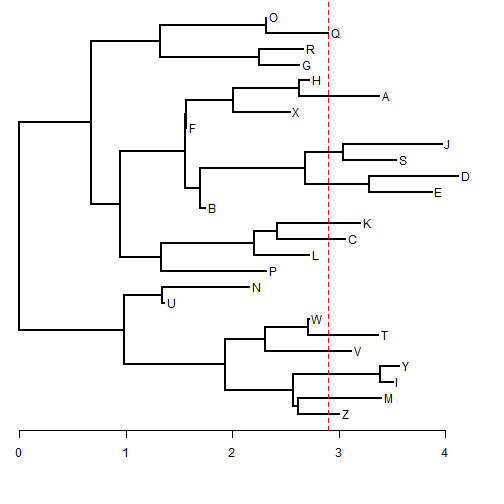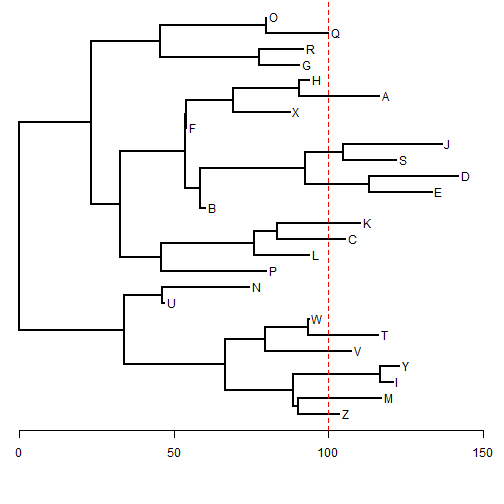## Tuesday, June 2, 2015

### Simple method to rescale tree to a particular mean tip height

Here's a simple trick to rescale a tree to have a particular mean height (if not ultrametric):

``````library(phytools)
## in this case we will use a random tree
tree<-rtree(n=26,tip.label=LETTERS)
plotTree(tree,mar=c(5.1,0.1,0.1,0.1))
axis(1)
## compute all tip heights
h<-sapply(1:Ntip(tree),nodeheight,tree=tree)
h
``````
``````##   2.998386 3.391986 3.502868 3.567715 3.110754 3.365106 2.714611
##   1.358671 2.152025 2.313791 2.715758 3.055251 3.199052 1.739875
##  3.869145 4.121823 3.534816 3.962804 1.561411 2.536063 3.378451
##  2.719116 2.628788 2.661650 2.900916 2.311506
``````
``````mean(h)
``````
``````##  2.898936
``````
``````lines(c(mean(h),mean(h)),par()\$usr[3:4],lty="dashed",col="red")
````````````## decide on new desired mean height
new.h<-100
## rescale tree
tree\$edge.length<-tree\$edge.length/mean(h)*new.h
h<-sapply(1:Ntip(tree),nodeheight,tree=tree)
h
``````
``````##   103.43058 117.00797 120.83289 123.06981 107.30675 116.08072  93.64164
##    46.86791  74.23498  79.81517  93.68118 105.39215 110.35264  60.01771
##  133.46776 142.18398 121.93495 136.69855  53.86151  87.48253 116.54105
##   93.79704  90.68114  91.81473 100.06830  79.73635
``````
``````mean(h)
``````
``````##  100
``````
``````plotTree(tree,mar=c(5.1,0.1,0.1,0.1))
axis(1)
lines(c(mean(h),mean(h)),par()\$usr[3:4],lty="dashed",col="red")
``````That's it.